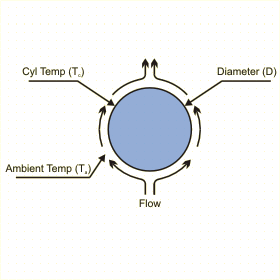Close

This page calculates the heat transfer coefficient, heat convected and heat radiated from an isothermal horizontal cylinder assuming natural convection. The convection calculation is based on Rayleigh number and is valid for Rayleigh numbers between 10-5 and 1012. The ends of the cylinder are assumed to be adiabatic.The heat loss from the cylinder (qc) is calculated as:

qc = hA (Tc - Ta)

Where h is the average heat transfer coefficient, A is the total area of the cylinder, Tc is the average temperature of the cylinder and Ta is the ambient fluid temperature. h is defined as:

h = Nu k / D

Where Nu is the Nusselt Number, k the conductivity of the fluid and D the diameter of the cylinder. The Nusselt number is calculated as:

Nu = { 0.60 + (0.387RaD1/6) / [1+(0.559/Pr)9/16](8/27) }2
where RaD = gB rho2Cp(Tc-Ta)D3 / kµ

For the above equations RaD is the Rayleigh number based on the diameter of the cylinder, g the gravitational acceleration, B the coefficient of thermal expansion, k the thermal conductivity, Pr the Prandtl number, a the thermal diffusivity and v the kinematic viscosity.

In addition, you must define the fluid properties at the film temperature Tf defined as follows, except for the density which is defined at the reference temperature of 20 C.

Tf = (Tp + Ta) / 2

The fluid density at the film temperature is automatically calculated from the following relationship based on the perfect gas law:

Rho = Rhoref (Tref +273) / (Tf + 273)

The radiative heat transfer is calculated as follows:

Qrad = Sigma A x E x(Tc4 - Ta4)
where Sigma, the Stefan-Boltzman constant = 5.678e-8
A is the cylinder area
E is the surface emissivity
Tc and  Ta are the plate and ambient temperatures respectively, in degrees Kelvin.

References

Churchill, S. W., and H. H. S. Chu, Correlating Equations for Laminar and Turbulent Free Convection from a Horizontal Cylinder, Int. J. Heat Mass Transfer, 18, 1049 1974.

Incropera, De Witt., Fundamentals of Heat and Mass Transfer, 3rd ed., John Wiley & Sons, p551, eq 9.34, 1990.